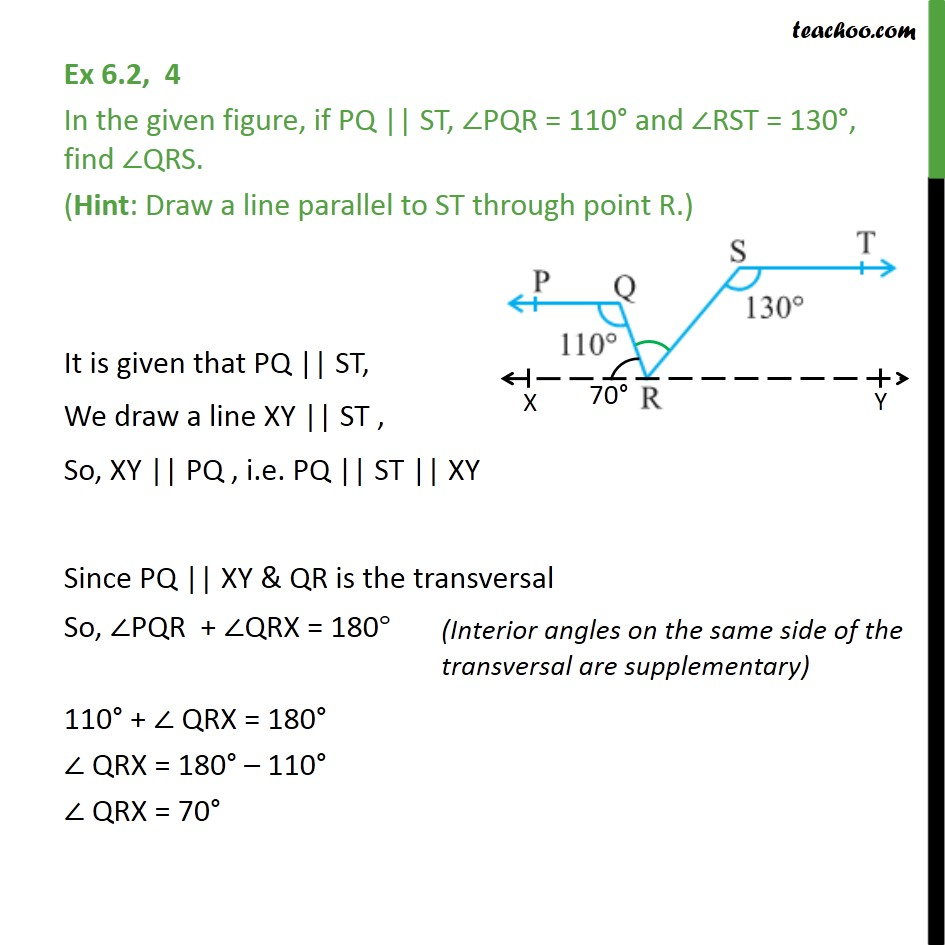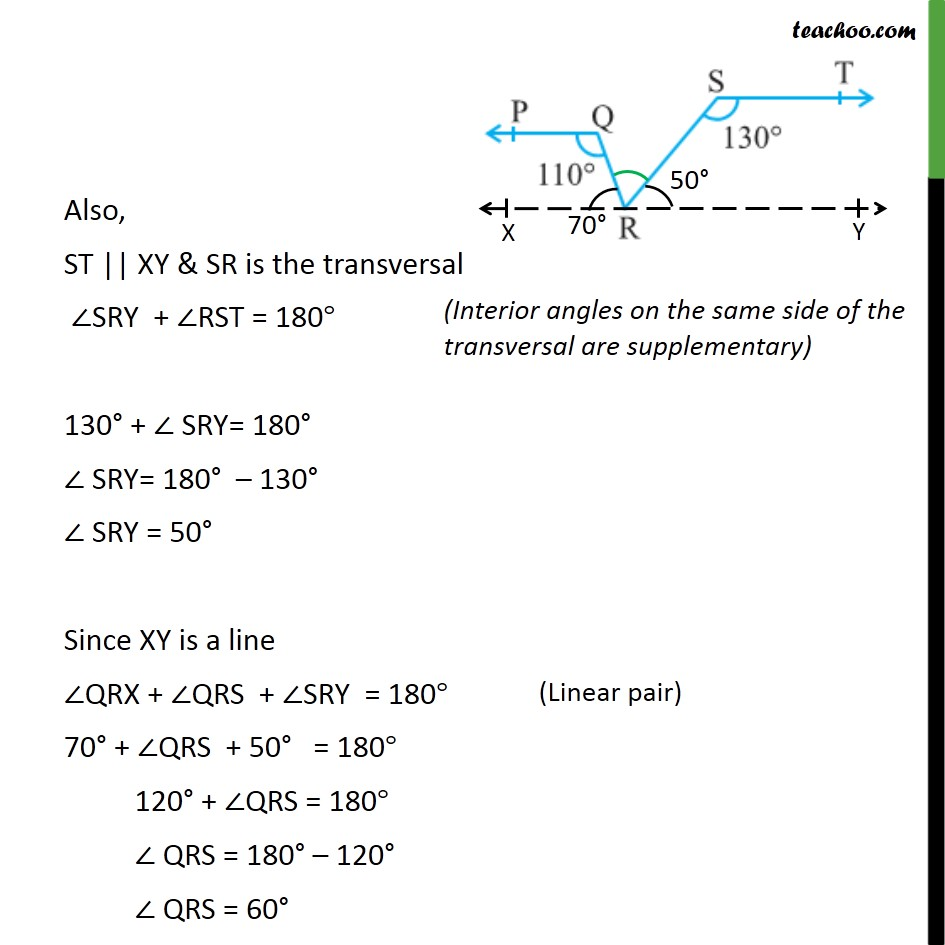1. Chapter 6 Class 9 Lines and Angles (Term 1)
2. Serial order wise
3. Ex 6.2

Transcript

Ex 6.2, 4 In the given figure, if PQ || ST, ∠PQR = 110° and ∠RST = 130°, find ∠QRS. (Hint: Draw a line parallel to ST through point R.) It is given that PQ || ST, We draw a line XY || ST , So, XY || PQ , i.e. PQ || ST || XY Since PQ || XY & QR is the transversal So, ∠PQR + ∠QRX = 180° 110° + ∠ QRX = 180° ∠ QRX = 180° – 110° ∠ QRX = 70° Also, ST || XY & SR is the transversal ∠SRY + ∠RST = 180° 130° + ∠ SRY= 180° ∠ SRY= 180° – 130° ∠ SRY = 50° Since XY is a line ∠QRX + ∠QRS + ∠SRY = 180° 70° + ∠QRS + 50° = 180° 120° + ∠QRS = 180° ∠ QRS = 180° – 120° ∠ QRS = 60°